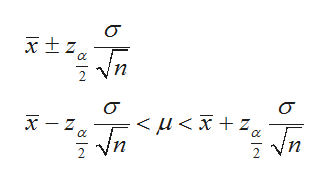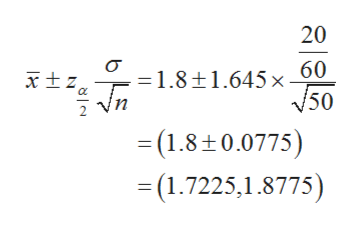A recent study showed that the modern working person experiences an average of 2.1 hours per day of distractions( phone calls, e-mails, impromptu visits,etc.).  A random sample of 50 workers for a large corporation found that these workers were distracted an average of 1.8 hours per day and the population standard deviation was 20 minutes.  Estimate the true mean population distraction time with 90% confidence, and compare your answer to the results of the study

Question

A recent study showed that the modern working person experiences an average of 2.1 hours per day of distractions( phone calls, e-mails, impromptu visits,etc.).  A random sample of 50 workers for a large corporation found that these workers were distracted an average of 1.8 hours per day and the population standard deviation was 20 minutes.  Estimate the true mean population distraction time with 90% confidence, and compare your answer to the results of the study

Step 1

Confidence interval:

The (1-α) % confidence interval for population mean µ, when σ is known is given by:help_outlineImage Transcriptionclose2 Vn 2 Vn 2 Vn 바투 바를 이들 fullscreen
Step 2

Computing the confidence interval for population mean:

A random sample of 50 workers for a large corporation fund that the workers were distracted an average of 1.8 hours per day and population standard deviation was 20 minutes (=20/60 hours). That is, n=50, x-bar = 1.8 and σ = 20/60.That is, the confidence level is 90%. Therefore, the significance level, α = 0.10.

From the EXCEL, using the formula, = NORM.INV(0.95,0,1), the critical value is 1.645.

Therefore the 90% confidence interval of the mean when the standard deviation is known is,help_outlineImage Transcriptionclose20 .=1.8±1.645×원 n 50 2 Vn (1.8+0.0775) (1.7225,1.8775) als fullscreen
Step 3

The 90% confidence interval for the true mean population distraction time is (1.7225,1.8775).

The recent study showed  that the modern working person experiences an av...

Want to see the full answer?

See Solution

Want to see this answer and more?

Our solutions are written by experts, many with advanced degrees, and available 24/7

See Solution
Tagged in

Other